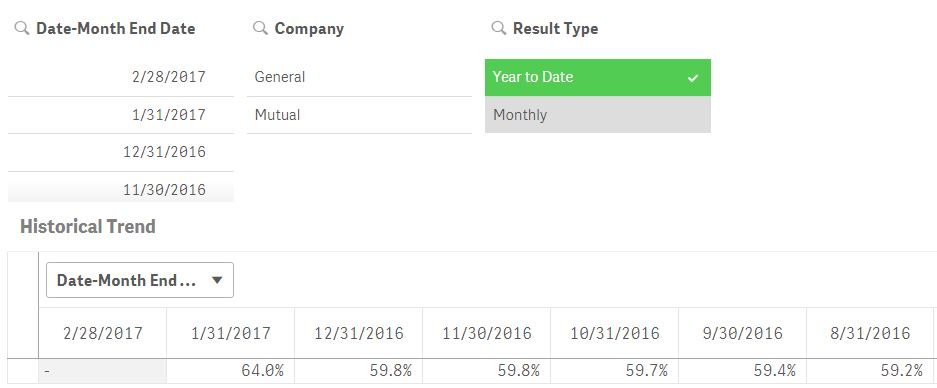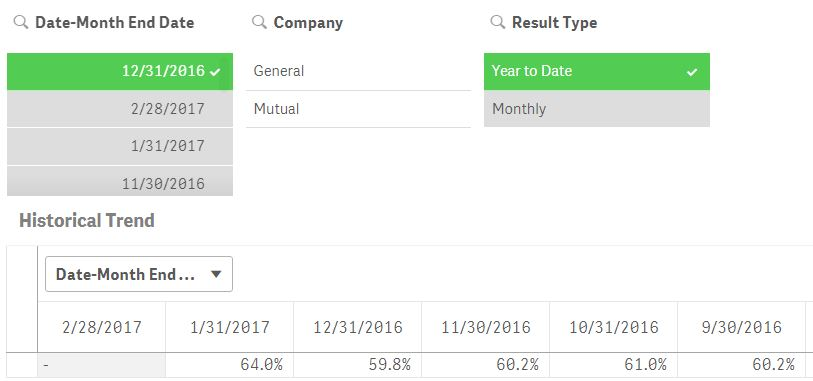# New to Qlik Sense

Discussion board where members can get started with Qlik Sense.

Announcements
Coming your way, the Qlik Data Revolution Virtual Summit. October 27-29. REGISTER
cancel
Showing results for
Did you mean:
HighlightedNot applicable

## Trying to use set analysis with measure expression to exclude filter from visual

I have a simple pivot table with date as the column and a measure (calculated ratio using an expression) as the row.  I have three filters (date, company, result type). The measure is calculated one of two ways depending on the result type selection (Year to Date or Monthly). I would like the pivot table to filter based on Company and Result Type but not the date. The following is the expression I am using for my measure:

IF([Result Type]='Year to Date', Sum({<[Date-Month End Date]=>}[YTD Losses Incurred])/Sum({<[Date-Month End Date]=>}[YTD Net Earned Premium]),Sum({<[Date-Month End Date]=>}[Losses Incurred])/Sum({<[Date-Month End Date]=>}[Combined Product Line.Net Earned Premium]))

If no date is selected with the filter pane, the pivot table produces the correct monthly and year to date results. However, if a date is selected from the filter pane, with year to date results, only the date that is selected has the correct year to date results all other months have the monthly results.

Any ideas on what I am missing in my measure expression that would cause this?  Thanks for the help!

Results with no date selected all year to date results are correct.Results when a specific date is selected - that date is correct in the pivot table but results for other dates in the pivot table are the monthly results (see 11/30/2016 it should be 59.8% as shown above).2 Replies
HighlightedMVP

Try this

IF(Only({<[Date-Month End Date]=>} [Result Type]) = 'Year to Date', Sum({<[Date-Month End Date]=>}[YTD Losses Incurred])/Sum({<[Date-Month End Date]=>}[YTD Net Earned Premium]),Sum({<[Date-Month End Date]=>}[Losses Incurred])/Sum({<[Date-Month End Date]=>}[Combined Product Line.Net Earned Premium]))

HighlightedNot applicable

That did it - thank you!